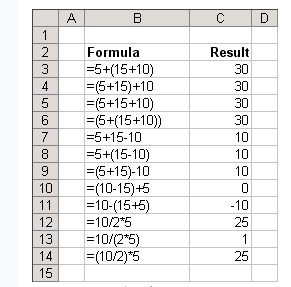#### The Importance of Parentheses in Excel Formulas

Parentheses are an important part of Excel formulas. They are used to group together certain parts of a formula and to indicate that these parts should be calculated first.

By using parentheses, you can control the order of operations in your formulas, ensuring that they are calculated in the way that you intended

## What do parentheses mean in Excel?

It is always a good habit to use the parentheses in Excel formulas, even if they are not compulsory for a formula, as they make it look good and more understandable.

We can put parentheses within parentheses and the rule works like this: the innermost parentheses are calculated first and then the outer ones.

When you enter brackets, you will notice that Excel tries to help you by making the matching parentheses bold as you pass over them with the mouse.

There must be a balance of parentheses as sometimes while creating a formula we enter the starting brackets and at the end we might lose count and forgot to enter the ending parentheses.

In that case, the Excel will prompt you with an error and it will be easy to know the issue. Excel will make a suggestion as to what it thinks the formula should be. You can accept the recommendation or press “No” to edit the formula yourself.

## How to use parentheses in Excel?

The first thing you need to remember is default order of calculations in mathematics:

1. Parentheses
2. Exponents
3. Division and multiplication

As you can see, putting brackets around variables changes the order of calculations and can change the results.

The following are some of the examples we can use to learn the use of brackets:

= 10 + (10*2) result: 30

If there were no parenthesis, the result could be different.

Now the inner bracket is calculated first and then the addition.

=((5*2) + (4 *2) )+ 2 = (10+8) + 2 = 20

How will it look without the use of parentheses:

= 5*2+4*2+2

Which is very confusing to look at.

Let us look at some more examples for more clarification:## Conditional Parentheses

Conditional parentheses are a powerful way to control the inclusion of certain calculations within a formula based on specific conditions. This technique is invaluable when you need to adjust calculations dynamically.

Suppose you have a dataset in which you want to calculate a bonus based on sales performance. The bonus is awarded if the sales exceed a certain target. You can use conditional parentheses to achieve this:

=IF(Sales > Target, (Sales – Target) * BonusRate, 0)

In this formula, if the sales exceed the target, the subtraction and multiplication are enclosed within parentheses, ensuring that the bonus calculation occurs. If not, the result is 0. Conditional parentheses allow you to conditionally include or exclude calculations within your formulas.